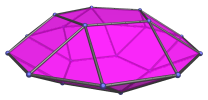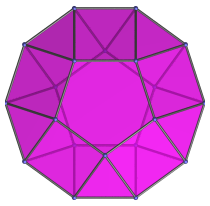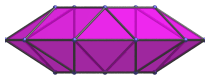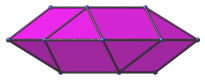# The Pentagonal Gyrobicupola

The pentagonal gyrobicupola is the 31st Johnson solid (J31). It has 20 vertices, 40 edges, and 22 faces (10 equilateral triangles, 10 squares, and 2 pentagons).The pentagonal gyrobicupola can be constructed by joining two pentagonal cupolae to each other at their decagonal face, such that the square faces of each cupola touch the triangular faces of the other at the edges. The gyro in the name refers to how the orientation of the two cupolae are rotated with respect to each other. Joining the two cupolae in ortho orientation produces the pentagonal orthobicupola (J30) instead.

A decagonal prism can be inserted between the two constituent cupolae to make an elongated pentagonal gyrobicupola (J39).

## Projections

Here are some views of the pentagonal gyrobicupola from various angles:

Projection DescriptionTop view.Front view.Side view.

## Coordinates

The Cartesian coordinates of the pentagonal gyrobicupola with edge length 2 are:

• (0, √((10+2√5)/5), 2√((3−φ)/5))
• (±φ, √((5−√5)/10), 2√((3−φ)/5))
• (±1, −√((5+2√5)/5), 2√((3−φ)/5))
• (±2φ, 0, 0)
• (±1, ±√(3+4φ), 0)
• (±φ2, ±√(2+φ), 0)
• (0, −√((10+2√5)/5), −2√((3−φ)/5))
• (±φ, −√((5−√5)/10), −2√((3−φ)/5))
• (±1, √((5+2√5)/5), −2√((3−φ)/5))

where φ = (1+√5)/2 is the Golden Ratio, approximately 1.61803.

Last updated 03 Sep 2018.